# Concrete Technology 2

## 20 Questions MCQ Test Mock test series of SSC JE Civil Engineering | Concrete Technology 2

Description
This mock test of Concrete Technology 2 for Civil Engineering (CE) helps you for every Civil Engineering (CE) entrance exam. This contains 20 Multiple Choice Questions for Civil Engineering (CE) Concrete Technology 2 (mcq) to study with solutions a complete question bank. The solved questions answers in this Concrete Technology 2 quiz give you a good mix of easy questions and tough questions. Civil Engineering (CE) students definitely take this Concrete Technology 2 exercise for a better result in the exam. You can find other Concrete Technology 2 extra questions, long questions & short questions for Civil Engineering (CE) on EduRev as well by searching above.
QUESTION: 1

### The amount of iron oxide in white Portland cement is limited to

Solution:

The process of manufacturing of white Portland cement is same as of OPC but the amount of iron oxide which is responsible for greyish colour is limited to less than 1%.

QUESTION: 2

### Air entrainment in the concrete increases

Solution:

Air entrainment is the intentional creation of tiny air bubbles in concrete. The air bubbles are created during mixing of the plastic (easy flowing) concrete, and most of them survive to be part of the hardened concrete. The primary purpose of air entrainment is to increase the durability of the hardened concrete, especially in climates subject to freeze-thaw; the secondary purpose is to increase workability of the concrete while in a plastic state. Air entrained concrete increases the workability of concrete without much increase in water-cement ratio.

QUESTION: 3

### The diameter of needle used in Vicat’s apparatus for the determination of initial setting time is prescribed as

Solution:

Vicat’s Apparatus is used to find out the consistency, initial setting time and final setting time of the cement. In the normal consistency test we have to find out the amount of water to be added to the cement to form a cement paste of normal consistency. Vicat's apparatus consists of an arrangement to hold the plunger of 10 mm diameter and two other needles which are made to freely fall into a mould filled with the cement paste and the amount of penetration of the needles of plunder can be noted using the vertical graduations from 0 mm to 50 mm.

For determination of initial setting time: The needle of diameter 1 mm is used.

QUESTION: 4

The initial and final setting times for ordinary Portland cement are approximately related as:
Where T and t are respectively final and initial setting times in minutes.

Solution:

The initial and final setting times for ordinary Portland cement are approximately related as:

T = 90 + 1.2t

where T and t are respectively final and initial setting times in minutes.

QUESTION: 5

The approximate ratio of strength of 15 cm cube to that of 15 cm x 30 cm concrete cylinder of the same concrete is

Solution:

The loading plates and the top/bottom surface of the concrete specimen offers some frictional resistance called as platen restraint which introduces shear stress at the top and bottom surfaces of the specimen and this effect diminishes as the distance between the platen surface increases. For this reason, standard concrete cube (height/diameter ratio = 1) specimen gives higher compressive strength then the cylindrical specimen (height/diameter ratio = 2).

Cube strength of concrete is nearly 1.25 times the cylindrical strength.

So, the ratio of strength of concrete cube to that of concrete cylinder is 1.25.

QUESTION: 6

UPV method in non-destructive testing for concrete is used to determine

1. Compressive strength

2. Existence of voids

3. Tensile strength

4. Static modulus of concrete

5. Dynamic modulus of concret

Solution:

Ultrasonic Pulse velocity method deals with the noting of time of travel of electronically generated mechanical pulse which is further used to analyse the velocity of pulse through the structure that represents its quality.

This test is used to establish uniformity of concrete, estimation of pulse modulus of elasticity, estimation of strength of concrete, determination of setting characteristics of concrete, estimation of crack depth, measurement of thickness of concrete pavement. This test calculates dynamic modulus of elasticity of concrete.

This test does not estimate tensile strength of concrete, so option a, b, d are wrong. So, correct answer is option c.

QUESTION: 7

For improvement of workability of concrete, the shape of aggregate recommended is

Solution:

If rounded aggregates are used in the concrete, it results in highly workable mixture as it offers minimum surface area to be lubricated by water cement paste. If angular aggregates are used, it will result in the high strength concrete while flaky aggregate in concrete neither possess strength nor any workability.

QUESTION: 8

The diameter of the pipeline used for transportation of concrete by pumps should not exceed:

Solution:

Generally, almost all pumped concrete is conveyed through 125 mm pipeline. General rule is that the pipe diameter should be between 3 to 4 times the largest size of aggregate. Concrete has been pumped to a height over 400 m and a horizontal distance of over 2000 m. But the diameter of the pipeline used for transportation of concrete by pumps should not exceed 30 cm.

QUESTION: 9

The process of removing the irregularities from the surface of concrete left after screeding is called

Solution:

The finishing of concrete surface is finally achieved by the following operations:

1. Screeding: it is the levelling operation that removes humps and hollows and give a true and uniform concrete surface.

2. Floating: It is the process of removing the irregularities from the surface of concrete left after screeding.

3. Trowelling: It is the final operation of finishing the concrete surface. It is performed where smooth and dense surface is required.

4. Curing: It is the process of hardening the concrete mixes by keeping its surface moist for a certain period, in order to enable the concrete to gain more strength.

QUESTION: 10

The durability of cement concrete is usually improved by the addition of:

Solution:

Durability of concrete may be defined as the ability of concrete to resist weathering action, chemical attack, and abrasion while maintaining its desired engineering properties. For a durable concrete, use of lowest w/c ratio is the fundamental requirement to produce dense and impermeable concrete. Lowering the water-cement ratio will lead to increase in the cement content thus increasing the durability. Mix must be designed to ensure cohesion and prevent segregation and bleeding. If cement is reduced, then at fixed w/c ratio the workability will be reduced leading to inadequate compaction. However, if water is added to improve workability, water / cement ratio increases and resulting in highly permeable material.

QUESTION: 11

The value of slump commonly adopted for the various concrete mixers are given below.

1. Concrete for road works: 20 to 28 mm

2. Ordinary RCC works: 50 to 100 mm

3. Columns retaining walls: 12 to 25 mm

4. Mass concrete: 75 to 175 mm

Which of the pairs given above are correctly matched?

Solution:

For concrete work low workable concrete is preferred.

∴ Slump value for concrete roads = (20 – 30) mm

Normal RCC work = (80 – 150) mm

Mass concrete = (20 – 50) mm

Columns and slabs = (40 – 50) mm

QUESTION: 12

To determined the modulus of rupture, the size for test specimen used is

Solution:

The modulus of rupture test employs a 150 × 150 × 700 mm plain concrete beam. The beam is simply supported and subjected to third-points loading until failure. Assuming a linear stress distribution across the cross-section, the theoretical maximum tensile stress reached in the extreme fibre is termed the modulus of rupture (fcr). It is obtained by applying the flexure formula.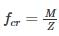Modulus of rupture is given by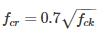QUESTION: 13

Workability of concrete can be measured using slump, compaction factor and Vee-bee time. Consider the following statements for workability of concrete:

(i) As the slump increases, the Vee-bee time increases.

(ii) As the slump increases, the compaction factor increases.

Which of the following is TRUE?

Solution:

As slump increases, Vee bee time decreases, as it becomes easier for concrete to flow.

It is clear from the below figure that as the slump increases, the compaction factor also increases.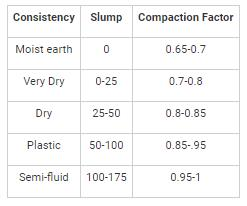QUESTION: 14

The upper limit of suspended particles in water for the preparation of concrete is:

Solution: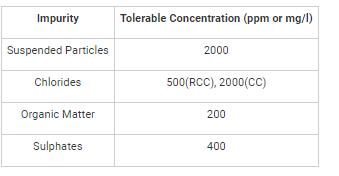QUESTION: 15

The ratio of bottom diameter to top diameter of the steel mould used for slump test is:

Solution:

Steel mould used to measure slump value is shown below:

The ratio of bottom mould to top mould is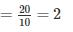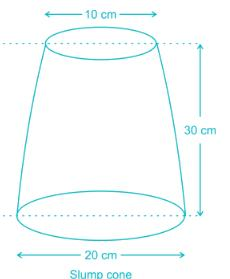QUESTION: 16

Generally the ratio of different ingredients (Cement Sand and aggregate) in concrete mix of grade M10 is:

Solution:

The nominal mixes of fixed cement-aggregate ratio (by volume) vary widely in strength and may result in under- or over-rich mixes. For this reason, the minimum compressive strength has been included in many specifications. These mixes are termed standard mixes.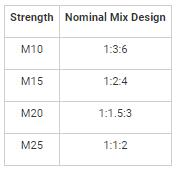IS 456-2000 has designated the concrete mixes into a number of grades as M10, M15, M20, M25, M30, M35 and M40. In this designation the letter M refers to the mix and the number to the specified 28 day cube strength of mix in N/mm2. The mixes of grades are as follows:

QUESTION: 17

Which one of the following is not required in concrete mix – design?

Solution:

As per IS 456 and IS 1343, the design of concrete mix should be based on the following factors:

• Characteristic compressive strength.
• Type of cement
• Maximum Nominal Size of Aggregate
• Water-Cement Ratio
• Workability
• Durability
• Degree of quality control
QUESTION: 18

To prevent segregation, the maximum height for placing concrete is:

Solution:

As per IS 456:2000, to prevent segregation, the maximum height for placing concrete is 150 cm.

Please refer IS 456:2000 section 13.2 " As a general guidance, the maximum permissible free fall of concrete may be taken as 1.5 m."

QUESTION: 19

Which factors comprise maturity of concrete?

Solution:

The strength of concrete depends both on temperature during curing and period of curing, and strength can be visualized as a function of period and temperature. The product (period x temperature) is called maturity of concrete. Here the temperature is taken from -10 degree Celsius which is the reasonable lowest value of temperature at which an appreciable increase in strength can take place. The maturity is measured in °C hours  or °C days.

QUESTION: 20

Bacterial concrete is one that:

Solution:

In bacterial concrete when cracks appear in a concrete structure and water starts to seep in through, the spores of the bacteria start microbial activities on contact with the water and oxygen. In the process of precipitating calcite crystals through nitrogen cycle the soluble nutrients are is converted to insoluble CaCO3 and remediate the cracks.